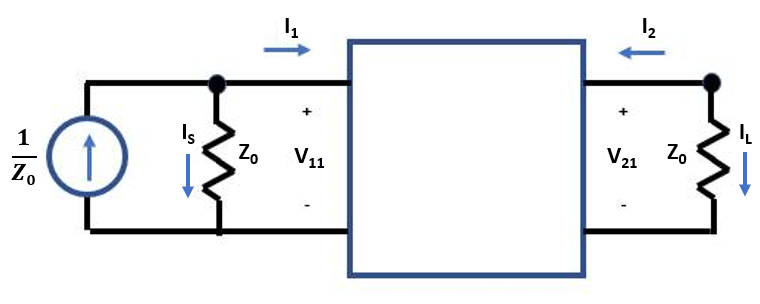# Extract S-Parameters from Circuit

This example uses the Symbolic Math Toolbox to explain how the RF Toolbox extracts two-port S-parameters from an RF Toolbox circuit object.

Consider a two-port network as shown in the figure 1that you want to characterize with S-parameters. S-parameters are defined as $\mathit{V}\text{\hspace{0.17em}}\mathit{–}\text{\hspace{0.17em}}\mathit{I}×{\mathit{Z}}_{0}=\text{\hspace{0.17em}}\mathit{S}\left(\mathit{V}\text{\hspace{0.17em}}+\text{\hspace{0.17em}}\mathit{I}×{\mathit{Z}}_{0}\right)$.Figure 1: Two-Port Network

To extract the S-parameters from a circuit into an `sparameters` object, the RF Toolbox terminates each port with the reference impedance ${\mathit{Z}}_{0}$. Then, the RF Toolbox independently drives each port j, with $\frac{1}{{\mathit{Z}}_{0}}$ and solves for the port voltages ${\mathit{V}}_{\mathit{ij}}$. Driving with current sources is the Norton equivalent of driving with a 1 V source and a series resistance of ${\mathit{Z}}_{0}$.

Measure the port voltage ${\mathit{V}}_{\mathit{ij}}$ at node i when node j is driven.

• If i $\ne$ j, the S-parameter entry ${\mathit{S}}_{\mathit{ij}}$ is simply twice the port voltage ${\mathit{V}}_{\mathit{ij}}$, and this is given using the equation ${\mathit{S}}_{\mathit{ij}}=2×{\mathit{V}}_{\mathit{ij}}$.

• The diagonal entries of S-parameters when $\mathit{i}=\mathit{j}$ are given using the equation ${\mathit{S}}_{\mathit{ij}}=2×{\mathit{V}}_{\mathit{ij}}-1$.Figure 2: Circuit Driven at Port 1 with Current Source

### Write Constitutive and Conservative Equations of Circuit

Circuits are represented in node-branch form in the RF Toolbox. There are four branches in the circuit represented in figure 2, one for the input port, two for the two-port `nport` object, and one for the output port. This means that the circuit has four branch current unknowns ${\mathit{I}}_{\mathit{S}}$, ${\mathit{I}}_{1}$, ${\mathit{I}}_{2}$, and ${\mathit{I}}_{\mathit{L}}$ and two node voltages ${\mathit{V}}_{11}$ and ${\mathit{V}}_{21}$. To represent the circuit described in figure 2 in node-branch form, you need four constitutive equations to represent the branch currents and two conservative equations to represent the node voltages.

```syms F IS I1 I2 IL V1 V2 Z0 syms S11 S12 S21 S22 nI = 4; % number of branch currents nV = 2; % number of node voltages % F = [Fconstitutive; Fconservative] F = [ V1 - Z0*IS V1 - Z0*I1 - S11*(V1+Z0*I1) - S12*(V2+Z0*I2) V2 - Z0*I2 - S21*(V1+Z0*I1) - S22*(V2+Z0*I2) V2 - Z0*IL IS+I1 I2+IL ]```
```F =  $\left(\begin{array}{c}{V}_{1}-\mathrm{IS} {Z}_{0}\\ {V}_{1}-{I}_{1} {Z}_{0}-{S}_{11} \left({V}_{1}+{I}_{1} {Z}_{0}\right)-{S}_{12} \left({V}_{2}+{I}_{2} {Z}_{0}\right)\\ {V}_{2}-{I}_{2} {Z}_{0}-{S}_{21} \left({V}_{1}+{I}_{1} {Z}_{0}\right)-{S}_{22} \left({V}_{2}+{I}_{2} {Z}_{0}\right)\\ {V}_{2}-\mathrm{IL} {Z}_{0}\\ {I}_{1}+\mathrm{IS}\\ {I}_{2}+\mathrm{IL}\end{array}\right)$```

### Jacobian Evaluation of Circuit

Use the `jacobian` function from the Symbolic Math Toolbox to compute the matrix of derivatives of the function `F` with respect to the six unknowns (four branch currents and two node voltages)

`J = jacobian(F,[IS; I1; I2; IL; V1; V2])`
```J =  $\left(\begin{array}{cccccc}-{Z}_{0}& 0& 0& 0& 1& 0\\ 0& -{Z}_{0}-{S}_{11} {Z}_{0}& -{S}_{12} {Z}_{0}& 0& 1-{S}_{11}& -{S}_{12}\\ 0& -{S}_{21} {Z}_{0}& -{Z}_{0}-{S}_{22} {Z}_{0}& 0& -{S}_{21}& 1-{S}_{22}\\ 0& 0& 0& -{Z}_{0}& 0& 1\\ 1& 1& 0& 0& 0& 0\\ 0& 0& 1& 1& 0& 0\end{array}\right)$```

### Solve S-Parameters of Circuit

Create a two-column right-hand side vector, `rhs`, to represent the driving of each port.

```syms rhs [nI+nV 2] syms x v S % Compute S-parameters of cascade rhs(:,:) = 0; rhs(nI+1,1) = 1/Z0; % rhs for driving input port rhs(nI+nV,2) = 1/Z0 % rhs for driving output port```
```rhs =  $\left(\begin{array}{cc}0& 0\\ 0& 0\\ 0& 0\\ 0& 0\\ \frac{1}{{Z}_{0}}& 0\\ 0& \frac{1}{{Z}_{0}}\end{array}\right)$```

To solve for the voltages, back solve the `rhs` with the Jacobian. The S-parameter matrix that MATLAB outputs represents the two-port circuit shown in figure 1.

```x = J \ rhs; v = x(nI+[1 nV],:); S = 2*v - eye(2)```
```S =  $\left(\begin{array}{cc}{S}_{11}& {S}_{12}\\ {S}_{21}& {S}_{22}\end{array}\right)$```